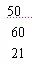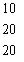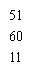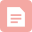# 以下不符合c语言语法的赋值语句是a.j++b.j=j=5,C语言单选题（五）

1.C语言结构体类型变量在程序执行期间(C)。

A.部分成员驻留在内存中

B.只有一个成员驻留在内存中

C.所有成员一直驻留在内存中

D.没有成员驻留在内存中

2.当定义一个结构体变量时，系统分配给它的内存是(D)。

A.结构中最后一个成员所需内存量

B.结构中第一个成员所需内存量

C.成员中占内存量最大者所需的容量

D.各成员所需内存量的总和

3.有以下枚举类型定义：enum a{sum=9,mon=-1,tue};则tue的值是(D)。

A.3

B.11

C.2

D.0

4.以下程序的输出结果是(D)。

struct  st

{int  x;

int *y;

}  *p;

int  dt = {10, 20, 30, 40};

structst  aa = { 50, &dt , 60,&dt , 60, &dt , 60, &dt};

main()

{ p =aa;

pritnf("%d\n", ++p -> x);

pritnf("%d\n", (++p) -> x);

pritnf("%d\n", ++(*p -> y) );

}

A.

B.C.D.5.应用缓冲文件系统对文件进行读写操作，关闭文件的函数名为(D)。

A.fwrite

B.close()

D.fclose()

6.以下叙述中错误的是(D)。

B.二进制文件打开后可以先读文件的末尾,而顺序文件不可以

C.在程序结束时,应当用fclose函数关闭已打开的文件

D.不可以用FILE定义指向二进制文件的文件指针

A.一个文件指针,指向要读的文件

B.一个存储区,存放要读的数据项

C.一个整数,代表要读入的数据项总数

8.C语言中，文件由(B)。

A.记录组成

B.由字符(字节)序列组成

C.由数据块组成

D.由数据行组成

9.以下不符合C语言语法的赋值语句是(C)。

A.a=b=5;

B.a=1,b=2

C.++j;

D.y=(a=3,6*5);

10.逻辑运算符两侧运算对象的数据类型(A)。

A.可以是任何类型的数据

B.只能是整型或字符型数据

C.只能是0或1

D.只能是0或非0正数

11.假设所有变量均为整型，则表达式(a=2,b=5,b++,a+b)的值是(D)。

A.6

B.7

C.2

D.8

12.用下列语句定义a，b，c，然后执行b=a、c='b'+b，则b，c的值是(C)。

long  a=0xffffff;

int  b; char  c;

B.-1和98

C.-1和97

D.0ffffff和0x61

13.下面四个选项中，均是不合法的用户标识符的选项是(C)。

A._123  temp int

B.A  P_0  do

C.b-a   goto  int

D.float  la0  _A

14.C语言中运算对象必须是整型的运算符是(D)。

A.=

B./

C.〈=

D.%=

15.不合法的八进制数是(D)

A.01

B.0

C.07700

D.028

16.下列表达式中，可作为C合法表达式的是(A)。

A.(3,2,1,0)

B.3/2/1/0

C.3=2=1=0

D.[3,2,1,0]

17.若有以下程序段，c3中的值是(D)

int  c1 = 1, c2 = 2, c3;

c3 = c1 / c2;

A.0.5

B.1

C.1/2

D.0

18.下列程序的输出结果是(D)。

main()

{

printf("%d\n",null);

}

A.-1

B.1

C.0

D.变量无定义

19.有以下程序

main()

{ int i = 10, j = 1;

printf("%d,%d\n",i--, ++j);

}

A.10,1

B.9,2

C.9,1

D.10,2

20.以下不符合C语言语法的赋值语句是(D)。

A.j=j+1;

B.j=j=5;

C.k=(2*4,k*4);

D.y=float(j);

21.C语言的if语句中,用作判断的表达式为(C)

A.关系表达式

B.逻辑表达式

C.任意表达式

D.算术表达式

22.有以下程序

main()

{

int a=5, b=4,c=3, d=2;

if(a>b>c)

printf("%d\n",d);

elseif((c-1 >= d) == 1)

printf("%d\n",d+1);

else  printf("%d\n",d+2);

}

A.2

B.4

C.编译时出错

D.3

23.int a=1,b=2,c=3;if(a>b)a=b; if(a>c)a=c;则a的值为(A)。

A.1

B.不一定

C.3

D.2

24.C语言的if语句嵌套时,if与else的配对关系是(C)

A.每个else与if的配对是任意的

B.每个else总是与最外层的if配对

C.每个else总是与它上面的最近的if配对

D.每个else总是与它上面的if配对

25.有以下程序段

int n=0,p;

do

{

scanf("%d",&p);

n++;

}while(p!=12345&&n<3);

A.p的值等于12345并且n的值大于等于3

B.p的值不等于12345并且n的值小于3

C.p的值不等于12345或者n的值小于3

D.p的值等于12345或者n的值大于等于3

26.从循环体内某一层跳出,继续执行循环外的语句是(A)

A.break语句

B.return语句

C.continue语句

D.空语句

27.t为int类型，进人下面的循环之前，t的值为0

while(t=1 )

{ ……}

A.循环控制表达式不合法

B.循环控制表达式的值为1

C.循环控制表达式的值为0

D.以上说法都不对

28.以下程序段的输出结果为(B)。

char c[]="abc";int  i=0;

do ;  while(c[i++]!='\0');

printf("%d",i-1);

A.ab

B.3

C.abc

D.2

29.若使用一维数组名作函数实参，则以下正确的说法是(A)。

A.必须在主调函数中说明此数组的大小

B.实参数组类型与形参数组类型可以不匹配

C.实参数组名与形参数组名必须一致

D.在被调用函数中,不需要考虑形参数组的类型

30.若有说明:inta[]={1,2,3,4,5,6,7};则a数组第一维的大小是(C)。

A.4

B.2

C.3

D.无确定值

31.以下对一维整型数组a的正确说明是(A)。

A.#defineSIZE 10  (换行)  int a[SIZE];

B.inta(10);

C.int n;scanf("%d",&n); int a[n];

D.intn=10,a[n];

32.以下对数组初始化错误的是：(C)

A.int a={{1,2,3},{4,5}}

B.int a={1,2,3,4};

C.int []={1,2,3,4,5,6};

D.int a[]={1,2,3,4,5,6};

33.以下函数调用语句中，含有的实参个数是(C)

func( (exp1, exp2), (exp3, exp4, exp5));

A.1

B.5

C.2

D.4

34.以下错误的描述是：函数调用可以(A)。

A.做为一个函数的形参

B.出现在执行语句中

C.做为一个函数的实参

D.出现在一个表达式中

35.下列定义不正确的是(D)。

A.#define PI 3.141592

B.#define S 345

C.static char c;

D.int max(x,y);  {  }

36.以下程序的输出结果是(C)

#define  MIN(x,y)   (x) < (y) ? (x) : (y)

main()

{   int i , j,k;

i = 10;  j = 15;

k = 10 *MIN(i, j);

printf("%d\n", k);

}

A.10

B.150

C.15

D.100

37.sizeof(float)是(B)。

A.一种函数调用

B.一个整型表达式

C.一个双精度型表达式

D.一个不合法的表达式

38.在说明语句:int*f();中，标识符f代表的是(A)。

A.一个返回值为指针型的函数名

B.一个用于指向函数的指针变量

C.一个用于指向一维数组的行指针

D.一个用于指向整型数据的指针变量

39.当运行以下程序时输入OPEN THE DOOR，则输出结果是(C)

#include

char fun(char  *c)

{  if(*c <= 'Z' && *c >= 'A' )

*c -= 'A' -'a';

return *c;

}

main()

{  char  s, *p = s;

gets(s);

while(*p)

{   *p = fun( p);

putchar(*p); p++;

}

putchar('\n');

}

A.oPEN tHE dOOR

B.Open The Door

C.open the door

D.OPEN THE DOOR

40.下面判断正确的是(D)。

A.char *a="china";等价于char *a;*a="china";

B.charstr={"china"};等价于char str;str[]={"china"};

C.charc="abc",d="abc";等价于char c=d="abc";

D.char *s="china";等价于char *s;s="china";博客

11-05948博客
C语言试卷选择判断题13
12-19736博客
01-24731博客
JAVA基础-面型对象测试题
04-095818博客

05-162397博客
c语言单选题大全,C语言单选题
05-171403博客

07-2062博客
C语言程序设计测试2 (附参考答案)

03-042万+博客
05-08791博客
c语言试题库之单选题
12-201017博客
c语言构成循环结构,C语言循环结构选择题
05-22872博客
c语言 练习题二
01-14153博客
{练习题}循环2
04-04377博客
c语言中有关for循环正确描述,2012年计算机等级二级C语言章节习题及答案5...
05-221258博客
20220312周测

04-22166博客

05-141315博客
C语言作业题(6)
01-171629博客
mysql第四章课外实践题答案_MySQL(四)--练习题
02-28282博客

05-162105

### “相关推荐”对你有帮助么？

•非常没帮助
•没帮助
•一般
•有帮助
•非常有帮助点击重新获取扫码支付余额充值